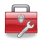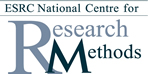# Using Statistical Regression Methods in Education Research# Module 2 - Simple Linear Regression

### Objectives

1. Know how to graph and explore associations in data
2. Understand the basis of statistical summaries of association (e.g. variance, covariance, Pearson's r)
3. Be able to calculate correlations and simple linear regressions using SPSS/PASW
4. Understand the formula for the regression line and why it is useful
5. See the application of these techniques in education research (and perhaps your own research!)## Start Module 2: Simple Linear Regression###### Get started with the basics of regression analysis.There are multiple pages to this module that you can access individually by using the contents list below. If you are new to this module start at the overview and work through section by section using the 'Next' and 'Previous' buttons at the top and bottom of each page. Be sure to tackle the exercises and the quiz to get a firm understanding.

This Module is also available as a downloadable PDF for those of you who prefer to read from a page. You do miss out on the glossary and some of the site's more interactive features though... Module 2: Simple Linear Regression#### Contents

2.1 Overview

2.3 Correlation

2.5 Simple Linear Regression

2.7 Using SPSS for Simple Linear Regression part 1 - running the analysis

2.8 Using SPSS for Simple Linear Regression part 2 - interpretting the output

Quiz

Exercise

 HomeModules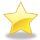Site Guide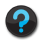Module 2 Contents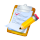Resources